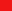###Author Topic: Wast Learniversity's Problem of the Day  (Read 2392 times)

####Wast##### Wast Learniversity's Problem of the Day
« on: April 26, 2012, 06:48:30 AM »
It's time for some learning! By "Problem of the Day", I mean an occasional problem when I feel like posting. Suggestions are welcome.

Quote
(Real Analysis) Show thatwhere C[a,b] is the space of continuous functions from [a,b] to the reals. Or, slightly more generally, show that for any f in L^\infty, the limit of the L^p norms of $f$ as p tends to infinity is the L^\infty norm of f.

####Wast##### Re: Wast Learniversity's Problem of the Day
« Reply #1 on: May 09, 2012, 12:08:35 AM »
Quote
(Real Analysis)
A topological space X is compact if every open cover of X has a finite subcover. A space is sequentially compact if every sequence in X has a convergent subsequence.

a) Show that a sequentially compact metric space has a countable base (a base for a topological space X is a collection of sets such that every open set in X can be expressed as a union of sets in this collection).

b) Show that sequential compactness implies compactness for a metric space X.

(Hint: For (a), for each integer n, construct a sequence of points that are each at least a distance 1/n apart. Argue that the construction terminates after a finite number of steps, and take the collection of all the points constructed.

For (b), take an open cover, find a countable subcover by base sets B_i, and let F_n be the complement of the union B_1 u B_2 u ... u B_n. Use sequential compactness to derive a contradiction if the F_n are non-empty.)

####Wast##### Re: Wast Learniversity's Problem of the Day
« Reply #2 on: May 14, 2012, 06:16:15 PM »
Quote
(Analysis) Let X be a complete metric space. Let diam(A) be the diameter of A, that is, sup(d(x,y)) over all x,y in A.

a) Show that if F_n is a nested sequence of closed sets with diam(F_n) -> 0, then the intersection of the F_n is non-empty.

b) Suppose V_n is a countable collection of dense open sets in X. Show that the intersection of the V_n is itself dense in X (this is a version of Baire's Theorem).

c) Let X be the space consisting of some sequence of points x_1,x_2,... and no other points. Define d(x_n,x_{n+i}) = 1 + /n for i>0 and set d(x_n,x_n) = 0. Show that (X,d) is a complete metric space. Find a sequence of nested closed sets whose intersection is empty.

####Wast##### Re: Wast Learniversity's Problem of the Day
« Reply #3 on: June 21, 2012, 10:53:32 PM »
Quote
(Hat Puzzle) A group of n bored children trapped inside on a rainy day wish for some entertainment to magically appear. They are excited to find that a magical Cat in a Hat arrives. Unfortunately, this Cat in the Hat is a mathematician, and forces them to act out a hat puzzle instead of having whimsical rhyming fun.

The Cat places a hat of either red or blue color on each child's head, such that the children can see every other hat but their own. Then the game commences, and the Cat stares awkwardly at them while they, in silence and without collaboration, write down either a guess for their hat color, or no guess at all.

Afterwards, each child checks the color of their hat. If at least one child has guessed, and no child is wrong, they win the round and are rewarded with some long division problems to experience the excitement of MATHS.

Suppose the children have time beforehand to determine an optimal strategy. What is the probability of success for such a strategy? Is it possible to make the probability of success arbitrarily close to one, given enough children?

(click to show/hide)
« Last Edit: June 21, 2012, 11:05:21 PM by Wast »

####Wast##### Re: Wast Learniversity's Problem of the Day
« Reply #4 on: June 21, 2012, 11:01:53 PM »
Quote
(A silly hat puzzle) Somewhere nearby the Hilbert Hotel is a prison where there are countably many prisoners. They are taking up too much space, so the warden decides to hold a competition in the form of a hat puzzle to give them a chance to earn their freedom (or at least to annoy them).

Each prisoner wears a hat with a real number on it, and the countably many prisoners stand on the positive number line on integer values, facing in the positive direction. The prisoner at position n can see all the hats and associated numbers at positions n+1 and above, but can see no other hats including his own. The arrangement is that the prisoners without collaboration must guess the color of their hat, and everyone who guessed correctly goes free.

The prisoners are given infinite time to collaborate beforehand, and are infinitely intelligent (as well as willing to work together despite a few of them inevitably remaining imprisoned). Thankfully, they also hold the power of the Axiom of Choice. Can they devise a strategy that will guarantee that all but finitely many escape?

####Wast##### Re: Wast Learniversity's Problem of the Day
« Reply #5 on: June 26, 2012, 07:34:25 PM »
Quote
(Transfinite Induction, and an open problem) The Axiom of Choice allows for some strange proofs. Consider transfinite induction, which extends the usual concept of induction that allows one to, for instance, induct over uncountable sets like the reals (with some caution). Here's a classic problem that uses transfinite induction. The original result is usually credited to Mazurkiewicz, and it's not known whether the Axiom of Choice is actually needed to solve it.

The problem: Find a subset of the plane that intersects every line in the plane exactly twice.
« Last Edit: July 09, 2012, 01:24:36 AM by Wast »